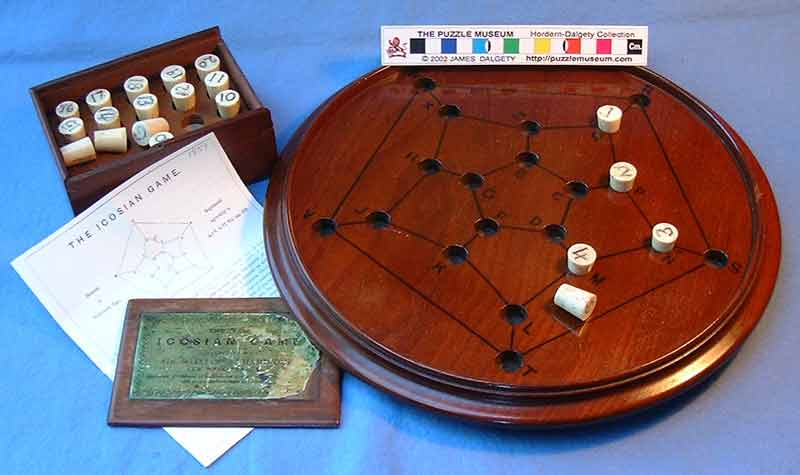$\newcommand{\set}{\{1,2,\dotsc,#1\,\}} \newcommand{\ints}{\mathbb{Z}} \newcommand{\posints}{\mathbb{N}} \newcommand{\rats}{\mathbb{Q}} \newcommand{\reals}{\mathbb{R}} \newcommand{\complexes}{\mathbb{C}} \newcommand{\twospace}{\mathbb{R}^2} \newcommand{\threepace}{\mathbb{R}^3} \newcommand{\dspace}{\mathbb{R}^d} \newcommand{\nni}{\mathbb{N}_0} \newcommand{\nonnegints}{\mathbb{N}_0} \newcommand{\dom}{\operatorname{dom}} \newcommand{\ran}{\operatorname{ran}} \newcommand{\prob}{\operatorname{prob}} \newcommand{\Prob}{\operatorname{Prob}} \newcommand{\height}{\operatorname{height}} \newcommand{\width}{\operatorname{width}} \newcommand{\length}{\operatorname{length}} \newcommand{\crit}{\operatorname{crit}} \newcommand{\inc}{\operatorname{inc}} \newcommand{\HP}{\mathbf{H_P}} \newcommand{\HCP}{\mathbf{H^c_P}} \newcommand{\GP}{\mathbf{G_P}} \newcommand{\GQ}{\mathbf{G_Q}} \newcommand{\AG}{\mathbf{A_G}} \newcommand{\GCP}{\mathbf{G^c_P}} \newcommand{\PXP}{\mathbf{P}=(X,P)} \newcommand{\QYQ}{\mathbf{Q}=(Y,Q)} \newcommand{\GVE}{\mathbf{G}=(V,E)} \newcommand{\HWF}{\mathbf{H}=(W,F)} \newcommand{\bfC}{\mathbf{C}} \newcommand{\bfG}{\mathbf{G}} \newcommand{\bfH}{\mathbf{H}} \newcommand{\bfF}{\mathbf{F}} \newcommand{\bfI}{\mathbf{I}} \newcommand{\bfK}{\mathbf{K}} \newcommand{\bfP}{\mathbf{P}} \newcommand{\bfQ}{\mathbf{Q}} \newcommand{\bfR}{\mathbf{R}} \newcommand{\bfS}{\mathbf{S}} \newcommand{\bfT}{\mathbf{T}} \newcommand{\bfNP}{\mathbf{NP}} \newcommand{\bftwo}{\mathbf{2}} \newcommand{\cgA}{\mathcal{A}} \newcommand{\cgB}{\mathcal{B}} \newcommand{\cgC}{\mathcal{C}} \newcommand{\cgD}{\mathcal{D}} \newcommand{\cgE}{\mathcal{E}} \newcommand{\cgF}{\mathcal{F}} \newcommand{\cgG}{\mathcal{G}} \newcommand{\cgM}{\mathcal{M}} \newcommand{\cgN}{\mathcal{N}} \newcommand{\cgP}{\mathcal{P}} \newcommand{\cgR}{\mathcal{R}} \newcommand{\cgS}{\mathcal{S}} \newcommand{\bfn}{\mathbf{n}} \newcommand{\bfm}{\mathbf{m}} \newcommand{\bfk}{\mathbf{k}} \newcommand{\bfs}{\mathbf{s}} \newcommand{\bijection}{\xrightarrow[\text{onto}]{\text{1--1}}} \newcommand{\injection}{\xrightarrow[]{\text{1--1}}} \newcommand{\surjection}{\xrightarrow[\text{onto}]{}} \newcommand{\nin}{\not\in} \newcommand{\prufer}{\mbox{prüfer}} \DeclareMathOperator{\fix}{fix} \DeclareMathOperator{\stab}{stab} \DeclareMathOperator{\var}{var} \newcommand{\inv}{^{-1}} \newcommand{\lt}{<} \newcommand{\gt}{>} \newcommand{\amp}{&}$

## Section2.3Hamiltonian cycles

We now introduce the concept of Hamiltonian walks. Though on the surface the question seems very similar to determining whether or not a graph is Eulerian, it turns out to be much more difficult.

###### Definition2.3.1

A graph is Hamiltonian if it has a closed walk that uses every vertex exactly once; such a path is called a Hamiltonian cycle

First, some very basic examples:

1. The cycle graph $C_n$ is Hamiltonian.
2. Any graph obtained from $C_n$ by adding edges is Hamiltonian
3. The path graph $P_n$ is not Hamiltonian.Figure2.3.2The Icosian game (from Puzzle Musuem) and its solution (from Wikipedia)

The term Hamiltonian comes from William Hamiltonian, who invented (a not very successful) board game he termed the "icosian game", which was about finding Hamiltonian cycles on the dodecahedron graph (and possibly its subgraphs)